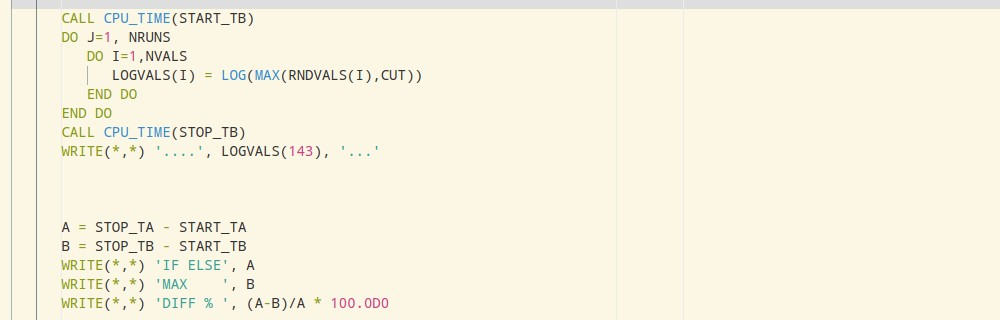# Timing a Fortran Program

Is it better to use MAX or IF/ELSE?This is a bit of Fortran you find in many simulations:

``````DO I=1,NVALS
IF (RNDVALS(I).GT.CUT) THEN
DTMP = RNDVALS(I)
ELSE
DTMP = CUT
END IF
LOGVALS(I) = LOG(DTMP)
END DO
``````

Or without the saving in the temporary `DTMP` variable. This does not look very nice, you have basically 5 lines to control the value before proceeding with calculations. An alternative is to push everything into the `MAX` intrinsic function:

``````DO I=1,NVALS
LOGVALS(I) = LOG(MAX(RNDVALS(I),CUT))
END DO
``````

This make the code cleaner, but it costs a `MAX` call at each iteration. The first rule in programming is that if you want to go fast, you need to do less. As I do not want to make my code significantly slower, I decided to benchmark the two different versions. So, I simulated the two cases with this sample code with calls to `CPU_TIME` to track the start/stop and a long enough run to gather reproducible values:

``````PROGRAM TEST

INTEGER, PARAMETER :: NVALS = 1000000
DOUBLE PRECISION RNDVALS(NVALS)
DOUBLE PRECISION LOGVALS(NVALS)
INTEGER I, J
INTEGER, PARAMETER :: NRUNS = 100
DOUBLE PRECISION, PARAMETER :: CUT = 0.001D0
DOUBLE PRECISION :: START_TA, STOP_TA, START_TB, STOP_TB
DOUBLE PRECISION DTMP, A, B

CALL RANDOM_NUMBER(RNDVALS)

CALL CPU_TIME(START_TA)
DO J=1, NRUNS
DO I=1,NVALS
IF (RNDVALS(I).GT.CUT) THEN
LOGVALS(I) = LOG(RNDVALS(I))
ELSE
LOGVALS(I) = LOG(CUT)
END IF
END DO
END DO
CALL CPU_TIME(STOP_TA)

CALL CPU_TIME(START_TB)
DO J=1, NRUNS
DO I=1,NVALS
LOGVALS(I) = LOG(MAX(RNDVALS(I),CUT))
END DO
END DO
CALL CPU_TIME(STOP_TB)

WRITE(*,*) '....', LOGVALS(143), '...'

A = STOP_TA - START_TA
B = STOP_TB - START_TB
WRITE(*,*) 'IF ELSE', A
WRITE(*,*) 'MAX    ', B
WRITE(*,*) 'DIFF % ', (A-B)/A * 100.0D0

END PROGRAM
``````

I compiled this test program with:

``````gfortran  -Ofast -funroll-loops test.for
``````

I did some runs with the `IF/ELSE` first and with the `MAX` first and basically, the results are the same. But a significant difference is coming from the `CUT` parameter.

• `CUT` is at `0.5D0`, the `IF/ELSE` approach is 40% faster.
• `CUT` is at `0.001D0`, the `MAX` approach is 2% faster.

Yes, the cut is the performance control. This is most likely because of the branch prediction. `MAX` is a function call and it costs most likely more to recover after a wrong prediction. Because the array if just full of random values between 0 and 1, in 50% of the cases, the prediction is false if the cut is at 0.5. If the cut is lower, that is the `MAX` is nearly always returning the `MAX` of one of the two values and always the same, it is faster.

Because in my thermodynamic code, the control on the value is the exceptional case, that is, we normally never trigger the `CUT`, it means that the branch prediction will be nearly always good and the `MAX` approach is not only as fast as the `IF/ELSE` case but nicer to read.

I was expecting the `MAX` call to always be slower.

Fluid Phase Equilibria, Chemical Properties & Databases